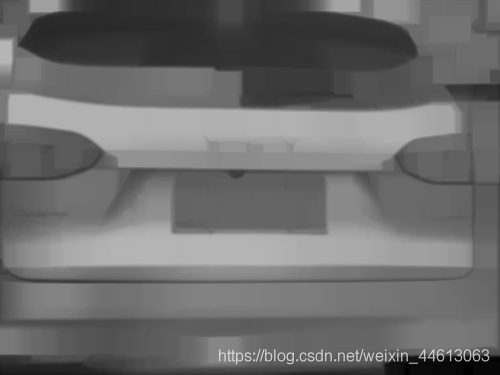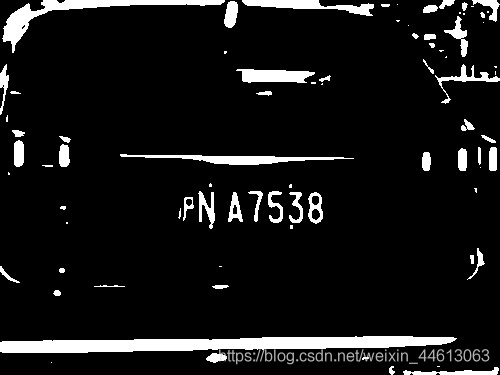# OpenCV实现车牌定位(C++)

更新时间：2020年11月27日 15:38:32   作者：Steven・简谈

```#include <iostream>
#include <opencv2/highgui/highgui.hpp>
#include <opencv2/imgproc.hpp>
#include <opencv2/imgproc/types_c.h>

using namespace std;
using namespace cv;

int main() {
// 读入原图
Mat gray_img;
// 生成灰度图像
cvtColor(img, gray_img, CV_BGR2GRAY);
// 在窗口中显示游戏原画
imshow("原图", img);
imshow("灰度图", gray_img);
waitKey(0);
return 0;
}```erode 操作也就是腐蚀操作，类似于卷积，也是一种邻域运算，但计算的不是加权求和，而是对邻域中的像素点按灰度值进行排序，然后选择该组的最小值作为输出的灰度值。

dilate 操作就是膨胀操作，与腐蚀操作类似，膨胀是取每一个位置邻域内的最大值。既然是取邻域内的最大值，那么显然膨胀后的输出图像的总体亮度的平均值比起原图会有所上升，而图像中较亮物体的尺寸会变大；相反，较暗物体的尺寸会减小，甚至消失。```// 得出轮廓
bool contour(Mat image, vector<vector<Point>> &contours, vector<Vec4i> &hierarchy) {
Mat img_gau, img_open, img_seg, img_edge;
// 高斯模糊
GaussianBlur(image, img_gau, Size(7, 7), 0, 0);
// 开运算
Mat element = getStructuringElement(MORPH_RECT, Size(23, 23));
morphologyEx(img_gau, img_open, MORPH_OPEN, element);
addWeighted(img_gau, 1, img_open, -1, 0, img_open);
// 阈值分割
threshold(img_open, img_seg, 0, 255, THRESH_BINARY + THRESH_OTSU);
// 边缘检测
Canny(img_seg, img_edge, 200, 100);
element = getStructuringElement(MORPH_RECT, Size(22, 22));
morphologyEx(img_edge, img_edge, MORPH_CLOSE, element);
morphologyEx(img_edge, img_edge, MORPH_OPEN, element);
findContours(img_edge, contours, hierarchy, RETR_TREE, CHAIN_APPROX_SIMPLE, Point());
return true;
}``````// 车牌轮廓点
Point2f(*choose_contour(vector<vector<Point>> contours)) {
int size = (int)contours.size();
int i_init = 0;
Point2f (*contours_result) = new Point2f[size];
for (int i = 0; i < size; i++){
// 获取边框数据
RotatedRect number_rect = minAreaRect(contours[i]);
Point2f rect_point;
number_rect.points(rect_point);
float width = rect_point.x - rect_point.x;
float height = rect_point.y - rect_point.y;
// 用宽高比筛选
if (width < height) {
float temp = width;
width= height;
height = temp;
}
float ratio = width / height;
if (2.5 < ratio && ratio < 5.5) {
contours_result[i_init] = rect_point;
contours_result[i_init] = rect_point;
i_init++;
}

}
return contours_result;
}

// 截取车牌区域
// 绘制方框
for (int i = 0; i < size; i++) {
Rect choose_rect(x, y, width, height);
Mat number_img = img(choose_rect);
imshow("车牌单独显示" + to_string(i), number_img);
}
}
imshow("绘制方框", img);
return 0;
}```

```int main() {
// 读入原图
Mat gray_img;
// 生成灰度图像
cvtColor(img, gray_img, CV_BGR2GRAY);
// 得出轮廓
vector<vector<Point>> contours;
vector<Vec4i> hierarchy;
contour(gray_img, contours, hierarchy);
// 截取车牌